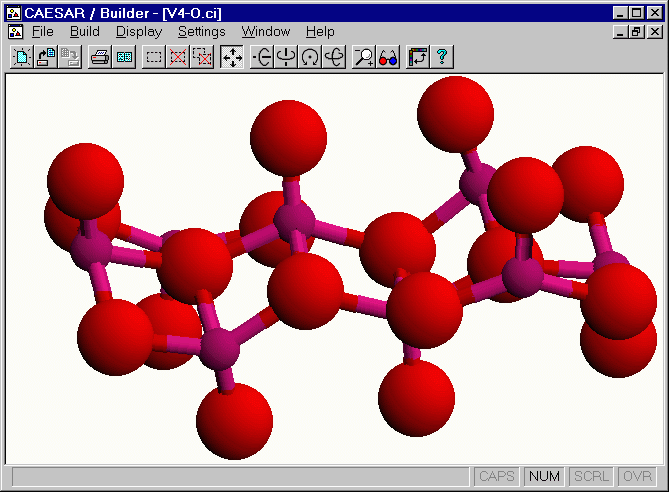# Unit cell of [H2N(CH2)4NH2)]V4O9

The [H2N(CH2)4NH2]V4O9 salt has two equivalent [V4O9]2- layers per unit cell. The following figure shows a perspective view of one [V4O9]2- layer, which was obtained by using the v4-o.CI file in the CAESAR\Examples\Chapter5\AV4O9 directory. This layer has four different vanadium atoms V(1), V(2), V(3) and V(4), each V atom is in a square-pyramidal coordinate, and the bond valence sum analysis 14 for each VO5 unit shows that the oxidation state of each V atom is V4+ (d1).Figure. Unit cell view of one layer of [H2N(CH2)4NH2]V4O9.

Go back to The Gallery